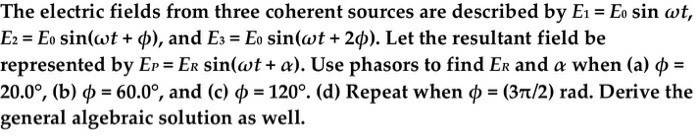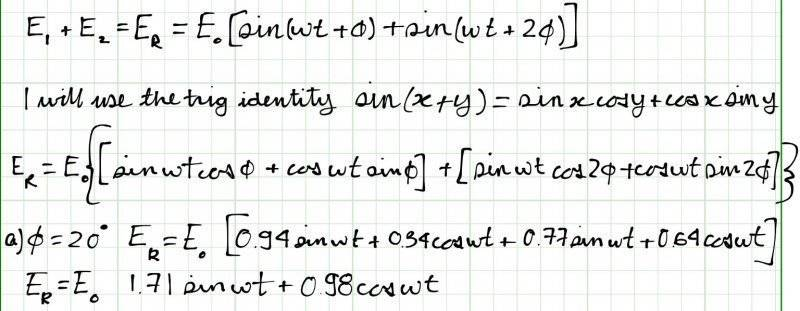# What is the resultant electric field?

• shanepitts

#### shanepitts

1. Homework StatementThe electric fields from three coherent sources are described by E1 = E0 sin omega t, E2 = E0 sin(omega t + phi), and E3 = E0 sin(omega t + 2phi). Let the resultant field be represented by EP = ER sin(omega t + alpha). Use phasors to find ER and alpha when (a) phi = 20.0 degree, (b) phi = 60.0 degree, and (c) phi = 120 degree. (d) Repeat when phi = (3pi/2) rad. Derive the general algebraic solution as well.

## Homework Equations

sin(a+b)=sinacosb+cosasinb

E1+E2=ER

## The Attempt at a SolutionMoreover, I am not sure how to calculate α and what is meant by deriving the general algebraic solution?[/B]

I believe that you are required to find the sum of all three electric fields. Also, I think you are supposed to get the answer by drawing the phasors and getting the answer from your drawing without using trig identities.

•shanepitts
I believe that you are required to find the sum of all three electric fields. Also, I think you are supposed to get the answer by drawing the phasors and getting the answer from your drawing without using trig identities.

After finding the phase via the phaser diagrams, how could I find the algebraic solution?Should I use the trig identities?

You should be able to get the phase ##\alpha## and the amplitude ##E_R## from the phasor diagram without using trig identities. You just need geometry and trig functions.

•shanepitts
You should be able to get the phase ##\alpha## and the amplitude ##E_R## from the phasor diagram without using trig identities. You just need geometry and trig functions.

I'm interpreting "find the general algebraic solution" as "find the general solution in terms of arbitrary ##\phi## and## E_0##. The phasor diagram shows how the result is the sum of three vectors. So, you can get the result by adding the three vectors either graphically or by method of components. The method of components is kind of messy and I think you would need to use some trig identities to get to a simple final result. But, if you draw the phasor diagram, then you can get the result by using just geometry and trig functions without needing trig identities.

•shanepitts